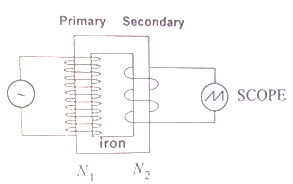# Problem: Consider an ideal transformer consisting of a primary coil of N 1 = 240 turns and a secondary coil of an unknown number of turns, N2 as shown below. The primary coil is connected to a function generator, which is set to produce a sinusoidal peak-to-peak voltage of 16 V. Using Using the oscilloscope, a student measures the peak-to-peak voltage difference across the secondary coil to be 56 V. What is N2? a) 70 b) 720 c) 840

89% (59 ratings)
###### Problem Details

Consider an ideal transformer consisting of a primary coil of N 1 = 240 turns and a secondary coil of an unknown number of turns, N2 as shown below. The primary coil is connected to a function generator, which is set to produce a sinusoidal peak-to-peak voltage of 16 V. Using Using the oscilloscope, a student measures the peak-to-peak voltage difference across the secondary coil to be 56 V. What is N2?

a) 70

b) 720

c) 840